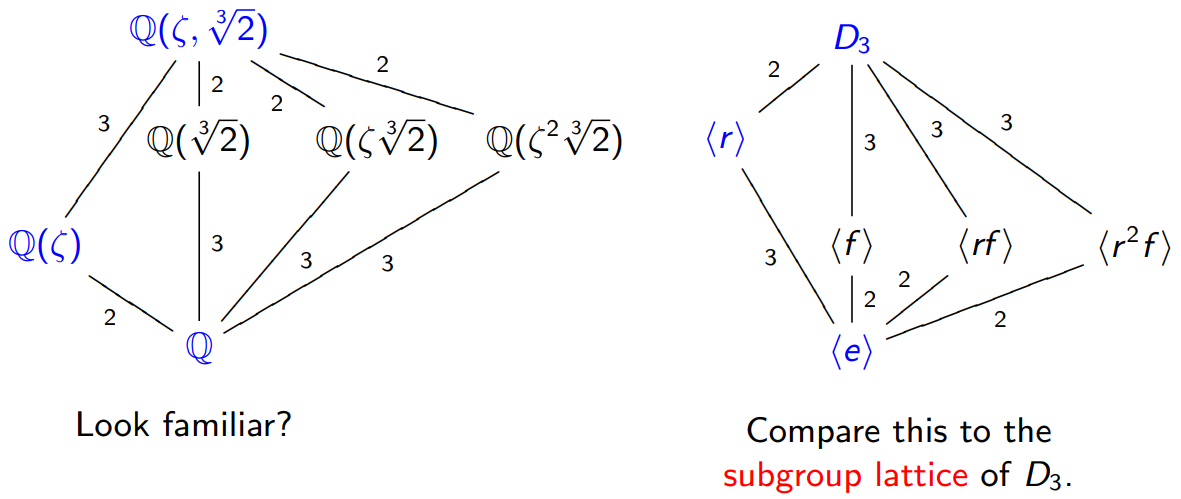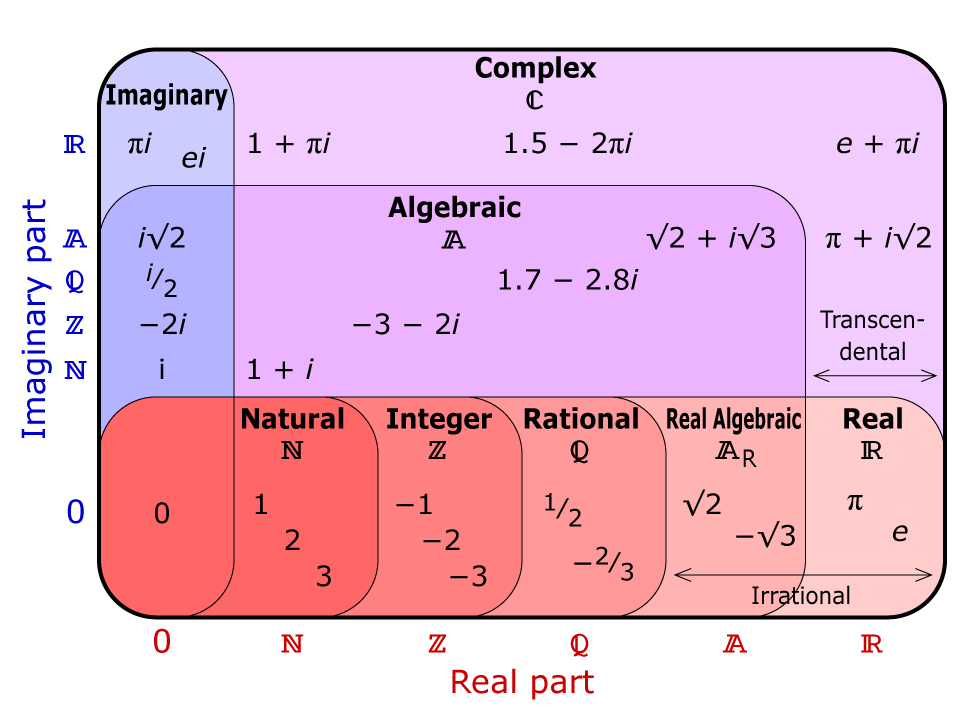# Galois Theory (Updating continuously)

Page content

## Good material

Visual Group Theory - Lecture 6 on YouTube is the best Galois theroy lecture.

You can fined slides of the lecture at below.

http://www.math.clemson.edu/~macaule/classes/m20_math4120/

• Kelin four group
• Hasse diagrams

complex number is algebraically closed. fundamental theorem of algebra Any plynomial with complex coefficients, having degree d, has at least one complex root.(Gauss)

## How to listen it

I listened 6.1 - 6.3 at the first day.

The famous result “there is no solution in radicals to general polynomial equations of degree five or higher with arbitrary coefficient” is revealed at 6.6. This theorem is called Abel-Ruffini theorem, but Galois proved it with geometry. This is why the Galois is famous.

should listen more

## Points

To do arithmetic, we need at least rational numbers.

### Definition of a field

• Two group structures are implemented. Both of the structures are abelian groups (addition on $F$ and multiplication on $F\setminus\{0\}$ ).
• The distributive low holds. $a(b+c) = ab + ac$.
• Examples: $\mathbb{Q}$, $\mathbb{R}$, $\mathbb{C}$, $\mathbb{Z}_{p}$ ($p$ is prime).

### Definition of field extension

• If $F$ and $E$ are fields with $F$ in $E$, $E$ is an extension of $F$.
• examples: $\mathbb{R}$ in $\mathbb{C}$, $\mathbb{Q}$ in $\mathbb{R}$
• Both sets have the same addition and multiplication.

#### For next stemp example 1

Think about the smallest extension field $F$ of $\mathbb{Q}$ that contains $\sqrt{2}$. It is called “$\mathbb{Q}$ adjoin $\sqrt{2}$”.

$$F = \{a+b\sqrt{2} | a,b \in \mathbb{Q}\}$$

#### For next stemp example 2

Think about the smallest extension field $F$ of $\mathbb{Q}$ that contains $i$. remarking as $\mathbb{Q}(i)$.

### Splitting field

• $\mathbb{Q}(\sqrt{2})$ contains all of the roots of $f(x)=x^2-2$, and $f(x)$ called splitting field.
• Because the elements of $\mathbb{Q}(\sqrt{2})$ split $f(x)$ into $(x+\sqrt{2})(x-\sqrt{2})$.
• Using large field, we can split the equation.
• $\mathbb{Q}(\sqrt{2})$ is the smallest to field $f(x)$.

### basis of extension

• we can think $\mathbb{Q}(\sqrt{2}, i)$ as a vector space whose basis is $1$, $\sqrt{2}$, $i$, and $\sqrt{2}i$.
• All elements of $\mathbb{Q}(\sqrt{2}, i)$ can be represented as $a+b\sqrt{2}+ci+d\sqrt{2}i$

### Spoiler(?)

Snipping from the slide.The numbers on the edge is the degree of polinomial whose roots need to adjoin.

### Field automorphism

• $\phi: F \rightarrow F$ for field $F$.
• $\phi$ is a bijection.
• $\phi(a+b) = \phi(a) + \phi(b)$ and $\phi(ab) = \phi(a)\phi(b)$. In other words, $\phi$ preserves the group structures.
• Example: for $\mathbb{Q}(\sqrt{2})$, $\phi: a+b\sqrt{2} \rightarrow a-b\sqrt{2}$ is a field automorphism.

Proposition:

If $\phi$ is an automorphism of an extension field $F$ of $\mathbb{Q}$, then $\phi(q)=q$ for all $q\in\mathbb{Q}$.

A proof overview (in the slide):

1. First, proof $\phi(1)=1$,
2. and extend using structure preserving properties.

### Galois group

Let $F$ be an extension field of $\mathbb{Q}$. The Galois group of $F$ is the group of automorphisms of $F$, denoted $\mathrm{Gal}(F)$.

#### Galois group example 1

$\mathrm{Gal}(\mathbb{Q}(\sqrt{2})) \cong C_2$.

$\cong$ is read “isomorphic to”, and the $C$ stands for Cyclic group.

#### Galois group example 2

$\mathrm{Gal}(\mathbb{Q}(\sqrt{2}, i)) \cong V_4$.

$V_4$ is Klein’s four group.

### Mysterious connection between “subfields of $F$” and “subgroups of $\mathrm{Gal}(F)$”.

Snipping from the slide. Analogy of field extention and dihedral group $D_3$. $\zeta = \frac{-1+\sqrt{3}i}{2}$The numbers on the edges are the number of cosets.

Reminder: coset, right coset and left coset, subgroup.

### Algebraic numbers

Definition:

A polinomial is a function $$f(x) = a_nx^n + a_{n-1}x^{n-1} + \cdots + a_2x^2 + a_1x + a_0$$

Let $F[x]$ denote the set of polynomials with coefficients in $F$, and call this “the set of polinomials over $F$.

Definition (from nLab): An algebraic number is a root of a polynomial with integer coefficients $\mathbb{Z}[x]$ (or, equivalently, with rational coeffients $\mathbb{Q}[x]$. It’s not obvious).

A number that is not algebraic over $\mathbb{Q}$ (e.g., $\pi$,$e$) is called transcendental.

Question: Can all algebraic numbers be expressed using radicals? Answer from Galois: No

Good picture from https://chalkdustmagazine.com/blog/complex-numbers-and-algebra/:#### Algebraically closed field

(Wikipedia) A field $F$ is algebraically closed if every non-constant polynomial in $F[x]$ (the univariate polynomial ring with coefficients in $F$) has a root in $F$.

### Fundamental theorem of algebra

The field $\mathbb{C}$ is algebraically closed.

Here is very good conceptional proof of the theorem.

https://youtu.be/shEk8sz1oOw?t=290

### Irreducibility of a polinomial

(From Wolfram MathWorld) A polynomial is said to be irreducible if it cannot be factored into nontrivial polynomials over the same field.

#### Eisenstein’s criterion

https://en.wikipedia.org/wiki/Eisenstein's_criterion

### Degree of a field extension

If $F \subseteq E$ are fields, then the degree of the extension, denoted $[E : F]$, is the dimension of $E$ as a vector space over $F$.

For example, $[\mathbb{Q}(i, \sqrt{2}) : \mathbb{Q}] = 4$

### Minimal polynomials

Let $r \notin F$ be algebraic. The minimal polynomial of $r$ over $F$ is the irreducible polynomial in $F[x]$ of which $r$ is a root. It is unique up to scalar multiplication.

Degree theorem: The degree of the extension $\mathbb{Q}(r)$ is the degree of the minimal polynomial of $r$.

### Splitting field

Let $f \in \mathbb{Z}[x]$ be a polynomial, with roots $r_1,\cdots,r_n$. The splitting field of $f$ is the field $\mathbb{Q}(r_1,\cdots,r_n)$.

### Galois group of a polinomial

The Galois group of a polynomial $f(x)$ is the Galois group of its splitting field, denoted $\mathrm{Gal}(f(x))$.

### Tower low

For any chain of field extensions, $F \subset E \subset K$, $$[K : F] = [K : E][E : F].$$

### Primitive elements

If $F$ is an extension of $\mathbb{Q}$ with $[F:\mathbb{Q}] < \infty$ then $F$ has a primitive element: some $\alpha\notin\mathbb{Q}$ for which $F=\mathbb{Q}(\alpha)$. (The proof is skipped :) )

### Galois group of a polinomial and roots of the polinomial

Let $K$ be a field and $F$ be an extension field of $K$. Let $f(x)\in K[x]$. Then every automorphism in $\mathrm{Gal}(F/K)$ permutes the roots of $f(x)$ in $F$.

Hense, if the degree of $f \in \mathbb{Z}[x]$ is $n$, then the Galois group of $f$ is a subgroup of $S_n$.

### Isomorphism and automorphism

Quickly referred here.

“Automorphism” is used in various field (category) of math. “Isomorphism” is an automorphism in Group theory.

### “One orbit theorem”

At this point, I tried to imagine map $\phi:\mathbb{C}\rightarrow\mathbb{C}$

• Suppose an irreducable polinomial $f(x)$ over $\mathbb{Q}$.
• This implicit the existence of an extension field $F$ over $\mathbb{Q}$.
• Suppose roots of the polinomial is $r_1$ and $r_2$.
• An element of the Galois group of $f(x)$ permutes $r_1$ and $r_2$.
• It is not obvious, but there is an isomorphism $\phi:\mathbb{Q}(r_1)\rightarrow\mathbb{Q}(r_2)$ that fixes $\mathbb{Q}$ and with $\phi(r_1)=r_2$.
• We know, “an element Galois group permutes the root”, but we didn’t know the existence of the permutation for arbitrary roots.

Pass the corollary so far.

### Normal subgroup

Given a group $G$. A group $N\subseteq G$ is called a normal subgroup if $gN = Ng,\ \forall g\in G$.

As an equivalent definitions: $gNg^(-1) = N \forall g\in N$

We denote this as $N\triangleleft G$

### Quotient group

https://en.wikipedia.org/wiki/Quotient_group